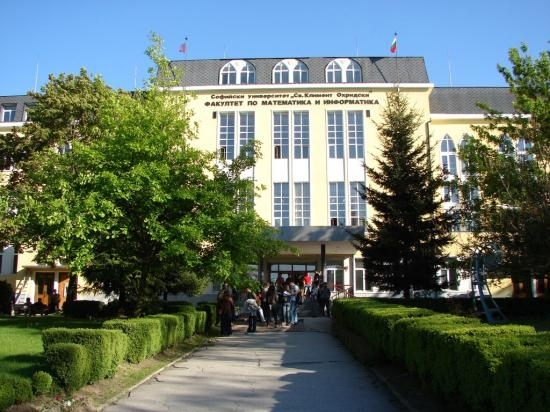# Master Programs at FMI, Sofia University, Evaluated as ECMI Model Master in Industrial MathematicsThe first specialization in Computational mathematics at FMI was created back in the 1959/60 academic year, inside the Department of Higher Analysis. It comprised of Numerical methods, Linear programming, Computers and programming, Theory of information as compulsory disciplines. Among the diploma works, given to the first students, graduating from this specialization, were “Modelling the harmonization of 8-bar melodies” and “Modelling of the belote game”. Thus, from its very beginning computational mathematics has been closely connected with mathematical modelling; what is more, it has been considered as a tool for mathematical modelling. A three-stage profiled education—Block A (3.5–4 years, Bachelor), Block B (1.5–2 years, Master), Block C (3 years, PhD)—was established at FMI in the 1970/71 academic year (let us note, 29 years before the Bologna process!!). Three of the branches of Block B were Computational mathematics, Mathematical modelling, and Operations research. During the years the names have been changed slightly, but the “mathematical modelling” has remained in the heart of all of the applied branches of education. This tradition has been kept till now and has determined the names of our Master Programmes.

MP Computational Mathematics and Mathematical Modelling (CMMM)

The Master Program Computational Mathematics and Mathematical Modelling (CMMM) was evaluated by the ECMI Educational Committee as ECMI Model Master in branch Technomathematics in 2012. The educational goal of CMMM is to provide students with a solid education in the following areas:

• creation and analysis of mathematical models of processes in Physics, Chemistry, Biology, Ecology and Engineering;
• development and studying of effective numerical methods and algorithms for solving the mathematical problems, obtained through the modelling;
• use of available software for scientific computations.

CMMM Master Program aims to prepare students to work in interdisciplinary research teams, to create mathematical models of real processes in at least one domain of science and engineering and to solve them by using contemporary numerical methods and high-performance computing. Four groups of courses make these goals achievable:

Mathematical modelling: Mathematical models and computational experiment, Mathematical modelling in Physics, Mathematical modelling in Biology, Hydrodynamics, Continuum mechanics, Non-linear mathematical models;

Contemporary numerical methods: Numerical methods for differential equations, Finite element method—algorithmic foundations, Numerical methods for system with sparse matrices, Parallel algorithms, Numerical integration;

Theory and analysis of numerical methods and continuous models: Theory of the finite difference schemes, Theory of the finite element methtods, Applied functional analysis, Sobolev spaces and applications in PDE;

Other tools: Spline-functions and applications, Wavelets and applications, Fourier transform, Wavelets and Signal processing, Computer graphics, Software for scientific computations.

The appropriate choice of courses from each group makes the CMMM master students capable to implement the full cycle of the Computational Experiment as a tool for investigating real-life problems: physical model–mathematical model–analytical investigation (as far as possible)–discrete model–algorithm–computer programme–numerical experiments–parametric investigation.

MP Mathematical Modelling in Economics (MME)

The Master Program Mathematical Modelling in Economics (MME) was evaluated by the ECMI Educational Committee as ECMI Model Master in branch Economathematics in 2012. The aim of MME is to develop student’s mathematical and computational skills to handle problems in business, finance and insurance giving them:

• knowledge of mathematical models in economics, finance and insurance;
• ability to handle amounts of data by numerical and statistical methods;
• skills in integrating Actuarial, Statistical and Financial techniques in modelling, take the right decisions in the area of insurance and financial practice.

MME Master Program’s goal is to stimulate the students to develop mathematical models of economics, finance and insurance and to solve them.  The students specialize in either Economics or Financial Mathematics and Actuarial Science. All students must pass the compulsory course in Econometrics. The elective courses are Optimal control, Applied general equilibrium, Macroeconomics, Game theory,  Nonlinear control systems, Financial mathematics, Stochastic analysis and applications, Numerical methods and applications, Time series, Mathematical risk theory, Life insurance, Insurance risk estimation, Credit risk.

Cooperation with insurance companies and the Central bank provides additional opportunities to analyze real models and data.

To create interest to applications of mathematics among the Bachelor students from all of the specialities at FMI and to attract them to the ECMI Model master programs, an elective course “Application of mathematics for modelling real processes” is offered for two years now. The Mathematical Modelling Seminar, obligatory for the Master students from CMMM and MME, where companies pose real-life problems to our students, and the participation in the ECMI Modelling weeks for five years now, create the spirit of our education.